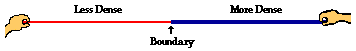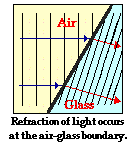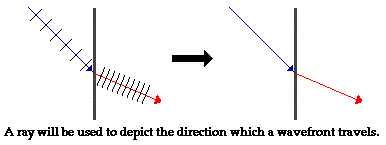Refraction and the Ray Model of Light - Lesson 1 - Refraction at a Boundary

# Boundary Behavior

A wave doesn't just stop when it reaches the end of the medium. Rather, a wave will undergo certain behaviors when it encounters the end of the medium. Specifically, there will be some reflection off the boundary and some transmission into the new medium. The transmitted wave undergoes refraction (or bending) if it approaches the boundary at an angle. If the boundary is merely an obstacle implanted within the medium, and if the dimensions of the obstacle are smaller than the wavelength of the wave, then there will be very noticeable diffraction of the wave around the object. The reflection, refraction, and diffraction of waves were first introduced in Unit 10 of The Physics Classroom tutorial. In Unit 11 of The Physics Classroom Tutorial, the reflection, refraction, and diffraction of sound waves was discussed. Since light is a wave, it too will undergo these same behaviors when it reaches a boundary between two media. The boundary behavior of light waves has already been introduced in Unit 12 of The Physics Classroom Tutorial. In this unit, we will focus on the refraction of light in great detail. We will explore the conceptual and mathematical principles governing the bending of waves as they cross the boundary between two media. To understand light refraction, we will need to back up a few steps and investigate the behavior of waves when they reach the end of a medium.

### Boundary Behavior for Waves on a Rope

Suppose that there is a thin rope attached to a thick rope, with each rope held at opposite ends by people. And suppose that a pulse is introduced by the person holding the end of the thin rope. If this is the case, there will be an incident pulse traveling in the less dense medium (thin rope) towards the boundary with a more dense medium (thick rope).Upon reaching the boundary, two behaviors will occur.

• A portion of the energy carried by the incident pulse is reflected and returns towards the left end of the thin rope. The disturbance that returns to the left after bouncing off the boundary is known as the reflected pulse.
• A portion of the energy carried by the incident pulse is transmitted into the thick rope. The disturbance that continues moving to the right is known as the transmitted pulse.

These two behaviors - reflection and transmission - were first introduced in Unit 10 of The Physics Classroom. In that unit, it was mentioned that the passage of the energy from the incident medium into the transmitted medium was accompanied by a change in speed and wavelength. In the case of a pulse crossing the boundary from a less dense medium into a more dense medium, the speed and the wavelength are both decreased. On the other hand, if a pulse crosses the boundary from a more dense medium into a less dense medium, the speed and the wavelength are both increased.### Refraction of Light Waves

The above discussion was limited to the behavior of a wave on a rope. But what if the wave is a light wave traveling in a three-dimensional medium? For example, what would happen if a light wave is traveling through air and reaches the boundary with a glass surface? How can the reflection and transmission behavior of a light wave be described? First, the light wave behaves like the wave on therope: a portion of the wave is transmitted into the new medium (glass) and a portion of the wave reflects off the air-glass boundary. Second, the same wave property changes that were observed for the wave on the rope are also observed for the light wave passing from air into glass; there is a change in speed and wavelength of the wave as it crosses the air-glass boundary. When passing from air into glass, both the speed and the wavelength decrease. Finally, and most importantly, the light is observed to change directions as it crosses the boundary separating the air and the glass. This bending of the path of light is known as refraction. A one-word synonym for refraction is bending. The transmitted wave experiences this refraction at the boundary. As seen in the diagram at the right, each individual wavefront is bent only along the boundary. Once the wavefront has passed across the boundary, it travels in a straight line. For this reason, refraction is called a boundary behavior. A ray is drawn perpendicular to the wavefronts; this ray represents the direction that the light wave is traveling. Observe that the ray is a straight line inside of each of the two media, but bends at the boundary. Again, refraction is a boundary behavior.

### The Ray Model of Light

In this unit, we will rely heavily on the use of rays to represent the direction in which light is moving. While we often think of light behaving as a wave, we will still find it useful to represent its movement through a medium using a line segment with an arrowhead (i.e., a ray) to depict the refraction of light. The ray is constructed in a direction perpendicular to the wavefronts of the light wave; this accurately depicts the light wave's direction. In this sense, we are viewing light as behaving as a stream of particles that head in the direction of the ray. The idea that the path of light can be represented by a ray is known as the ray model of light. The same ray model was utilized in Unit 13 of The Physics Classroom Tutorial to discuss the reflection of light waves.Next Section:
Jump To Next Lesson:

Follow Us